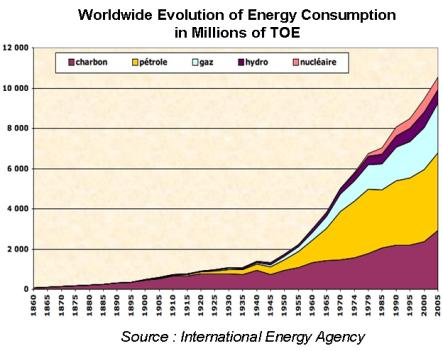Chargement...

# 2) Power of human origin 2) Power of human origin

2) Power of human origin

Before we study the Global Warming issue, as a “warm up” we are going to verify if the order of magnitude of the worldwide power of energy production which appears to be 1.5 x E13 [W] in 2008 is correct.

In order to perform this verification, we are going to use the worldwide consumption balances, considering all uses and all energies.>

The ton of oil equivalent (TOE) is a consumption unit that corresponds to the quantity of heat released by the combustion of a ton of oil.

One TOE = 11,600 [kWh]

## Question

Given the indications above, and given that one [kWh] corresponds to 3,600 [kJ], compute in [W] the worldwide energetic power produced in 2005.

The worldwide energetic power produced in 2005 is: 1.4 x E13 [W]

Explanation:
According to the I.E.A. graph, worldwide energy consumption in 2005 amounted to 10,500,000,000 tons of oil equivalent (TOE)

One TOE corresponds to 11,600 [kWh]
One kWh corresponds to 3,600,000 [J]
The worldwide energy consumption in 2005 was therefore:
Q= 10,500,000,000 x 11,600 x 3,600,000 = 4.38 x E20 [J]
The corresponding power was:
P = Q/t

P = 4.38 x E20 / 365 x 24 x 3,600 = 1.4 x E13 [W]Considering the worldwide energy consumption, the power produced in 2005 was of 1.4 x E13 [W], the estimation of 1.5 x E13 [W] in 2008 is therefore coherent.